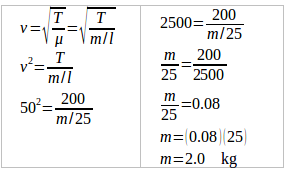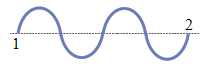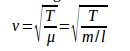# Speed of the mechanical waves – problems and solutions

1. The speed of the transverse wave on a 25 meters rope is 50 m/s. The tension force of the rope is 200 N. Determine the mass of rope.

A. 0.08 kg

B. 0.5 kg

C. 0.8 kg

D. 2.0 kg

Known :

Length of rope (l) = 25 m

Speed of transverse wave (v) = 50 m/s

Tension force of rope (T) = 200 N

Wanted: Mass of rope (m)

Solution :

This problem can be solved using the formula of the wave propagation formula on the rope.2. On the surface of the lake floats two pieces of wood, the distance between them is 6 m. The waves propagate on the surface of the lake. When the first wood has moved up and down three times, the second wood just moved up and down once. If every 2 seconds both kinds of wood move up and down three times, the wave propagation is …

A. 4.5 m/s

B. 3.0 m/s

C. 2.0 m/s

D. 1.5 m/s

Known :

Distance between both woods (l) = 6 meters

The first timber has moved up and down three times; the second wood just moved up and down once.

Frequency (f) = 3 / 2 = 1.5 hertz

Wanted: Speed of wave (v)

Solution :

When the first wood moves up and down once, the wavelength 1 travels to the second wood. When the first wood moves up and down twice, the wavelength 2 travels to the second wood. When the first wood moves up and down three times, the wavelength one has arrived at the second wood position, so that at the same time the second wood moves up and down once.Thus, the distance between the first wood and the second wood is two wavelengths (2 λ), as shown in the picture on the side.

Distance of 1 wavelength (λ) = 6 / 2 = 3 meters

The speed of the wave propagation (v):

v = f λ = (1.5 hertz)(3 meters)

v = 4.5 m/s

3. Factors that accelerate the propagation of waves on the strings are…

(1) extend the wire

(2) magnifying the wire mass

(3) increase the wire tension

(4) enlarge the wire size The correct statement is…

A. (1), (2), (3) and (4)

B. (1), (2), and (3)

C. (3) and (4)

D. (3) only

Solution :

The formula of the speed of the wave propagation on the strings:v = speed of the wave propagation on the strings

T = tension force of strings

μ = density of the string

m = mass of the string

l = length of string

4. On a rope propagates a wavelength rope with a frequency of 50 Hz and a wavelength of 2 m. Period and velocity of the wave are…

A. 0.02 seconds and 50 ms-1

B. 0.02 seconds and 100 ms-1

C. 0.04 seconds and 50 ms-1

D. 0.5 seconds and 100 ms-1

Known :

Frequency (f) = 50 Hz

The wavelength (λ) = 2 meters

Wanted: Period (T) and speed of the wave (v)

Solution :

Period :

T = 1/f = 1/50 Hz = 0.02 seconds

The speed of wave :

v = f λ = (50 1/second)(2 meters) = 100 meters/second

5. A cork on the surface of water moves up and down due to the surface water waves. The time required from a crest to next crest is 1.2 seconds. If on the water surface, the distance between two crests is 10 cm, then determine the speed of the wave propagation.

A. 12 ms-1

B. 12 cm s-1

C. 8,3 ms-1

D. 8,3 cm s-1

Known :

The time required from crest to the next crest = the time interval for one wavelength = period (T) = 1.2 seconds.

Distance between two crests = distance of 1 wavelength (λ) = 10 cm

Wanted : Speed of the wave propagation (v)

Solution :

Speed of the wave propagation :

v = f λ = λ / T = 10 cm / 1.2 seconds = 8.3 cm/second = 8.3 cm.s-1